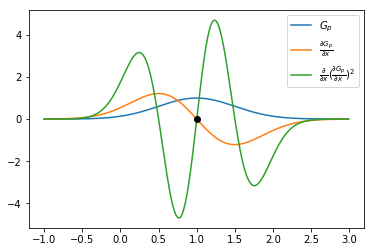In :
import sys
sys.path.append('/usr/local/lib')

In :
import molgrid
import torch
import torch.nn.functional as F
import matplotlib.pyplot as plt
import numpy as np
%matplotlib inline


Consider the following example where we have an atom of radius 1.0 on a grid: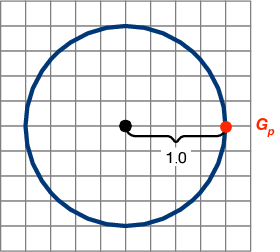In :
gmaker = molgrid.GridMaker(resolution=0.5,dimension=6.0)


## Density Functions (Forward)¶

We will focus on the calculation of $G_p$. As this is on the radius, this is right at the switch-over from the Gaussian density to the quadratic decay term (the value and derivative are the same here).

The Gaussian grid density $g_{\mathit{G}}$ and quadratic grid density $g_{\mathit{q}}$ as a function of the distance $d$ from atom center is:

\begin{align} g_{\mathit{G}}(d) & = e^{-2\frac{d^2}{r^2}} \\ g_{\mathit{q}}(d) & = 4e^{-2}\frac{d^2}{r^2}-12e^{-2}\frac{d}{r}+9e^{-2} \end{align} For the remainder I will set $r = 1$ to match our example and simplify the math, but it needs to be put back in during implementation.

\begin{align} g_{\mathit{G}}(d)&= e^{-2d^2} \\ \frac{\partial g_{\mathit{G}}}{\partial d} &= -4de^{-2d^2}\\ \frac{\partial^2 g_{\mathit{G}}}{\partial d^2} &= 16d^2 e^{-2d^2} - 4e^{-2d^2} \end{align}

\begin{align} g_{\mathit{q}}(d) &= 4e^{-2}d^2-12e^{-2}d+9e^{-2} \\ \frac{\partial g_{\mathit{q}}}{\partial d} &= 8e^{-2}d-12e^{-2} \\ \frac{\partial^2 g_{\mathit{q}}}{\partial d^2} &= 8e^{-2} \\ \end{align}

Note that: \begin{align} g_{\mathit{q}}(1.5) &= 0 \\ g_{\mathit{G}}(1) & = g_{\mathit{q}}(1) = e^{-2} \\ g_{\mathit{G}}'(1) & = g_{\mathit{q}}'(1) = -4e^{-2} \end{align} but \begin{align} g_{\mathit{G}}''(1) = 12e^{-2} \ne g_{\mathit{q}}''(1) = 8e^{-2} \end{align}

This is a concern in training models that use the second derivative as there is a discontinuity (similar to with ReLUs), but it isn't possible to fit a quadratic to have the correct values and first and second order derivatives.

In :
coords = torch.zeros(1,3,dtype=torch.float32,requires_grad=True)
types = torch.ones(1,1,dtype=torch.float32)

outgrid = molgrid.Coords2GridFunction.apply(gmaker, (0,0,0), coords, types, radii)

In :
outgrid

Out:
tensor([0.0000, 0.0000, 0.0000, 0.0000, 0.1353, 0.6065, 1.0000, 0.6065, 0.1353,
0.0000, 0.0000, 0.0000, 0.0000], grad_fn=<SelectBackward>)

The numbers above correspond to a line through the middle of the grid.

In :
np.exp(-2) # g(1)

Out:
0.1353352832366127

## Training (Backward)¶

Without loss of generality, we will consider exactly one grid point, $G_p$. The input to our network is an atom center, $(x,y,z)$ and we are going to compute the density value at $G_p$ as our loss.

\begin{align} L(x,y,z) &= g(\mathit{dist}(x,y,z,G_p)) \end{align}

The distance function is: \begin{align} \mathit{dist}(x,y,z,G_p) = \sqrt{(G_{p_x}-x)^2+(G_{p_y}-y)^2+(G_{p_z}-z)^2} \end{align} For simplicity, we will reduce this to the one dimension case. Our example is $(x,y,z) = (0,0,0)$ and $G_p = (1,0,0)$ so we will only consider x. We want to know how changing $x$ while change the value of $L(x)$. \begin{align} L(x) &= g(\mathit{dist}(x,G_{p_x})) \\ \frac{\partial L}{\partial x} &= \frac{\partial L}{\partial d} \frac{\partial d}{\partial x}, \mathrm{where} \label{lieb} \\ \frac{\partial L}{\partial d} &= g'(d) \\ \frac{\partial d}{\partial x} &= sign(x-1) \end{align} Thus, for our example \begin{align} L'(0) = g'(1)(-1) = 4e^{-2} \end{align} This is saying if I increase x, the output value (the density) will increase, which is correct.

In :
gp = outgrid
gp

Out:
tensor(0.1353, grad_fn=<SelectBackward>)

Pytorch correctly calculates the gradient to be $4e^{-2} = 0.54134$

In :
Lg = torch.autograd.grad(gp,coords)
Lg

Out:
(tensor([[0.5413, 0.0000, 0.0000]]),)
In :
np.exp(-2)*4

Out:
0.5413411329464508

Now imagine what we're really interested in is a loss function on the gradient itself $\mathcal{L}$. Note I'm specifically giving this a different symbol to emphasize that this is just another function. For example, if you had some true'' gradient $X$ you wanted to compare with: \begin{align} \mathcal{L}(x) &= (L'(x)-X)^2 \end{align} Then you want to know how a change in $x$ will effect $\mathcal{L}$.

For an initial simple case, let's again assume a fixed $G_p = (0,0,1)$ and calculate in terms of the distance $d$ from the atom center. First we consider the quadratic side of the gridding function, $g = g_q$. \begin{align} \frac{\partial \mathcal{L}}{\partial d} &= 2(L'(d)-X) L''(d) \\ L(d) &= 4e^{-2}d^2-12e^{-2}(d)+9e^{-2} \\ L'(d) &= 8e^{-2}d-12e^{-2} \\ L''(d) & = 8e^{-2} \end{align} This is expected - the second derivative of a 2nd order polynomial should be constant. For the case where $g = g_G$: \begin{align} L(d) &= e^{-2(d)^2} \\ L'(d) &= -4(d)e^{-2(d)^2} \\ L''(d) &= 16 \, d^{2} e^{\left(-2 \, d^{2}\right)} - 4 \, e^{\left(-2 \, d^{2}\right)} \\ \end{align}

Let's get a little visual inspiration.

In :
x = np.arange(-2,2.1,.1)
plt.plot(x,np.exp(-2*x**2),label='L(d)')
plt.plot(x,-4*x*np.exp(-2*x**2),label="L'(d)")
plt.plot(x,16*x**2*np.exp(-2*x**2)-4*np.exp(-2*x**2),label="L''(d)")  # this is NOT the square of L'
plt.plot(,[-4*np.exp(-2)],'ok')
plt.legend(loc='best');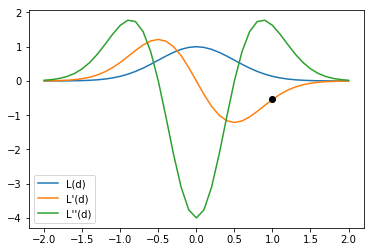This means the gradient of our loss $\mathcal{L}$ at $d = 1$ is: \begin{align} \mathcal{L}'(1) &= 2(L'(1)-X) L''(1) \\ \mathcal{L}'(1) &= 2(8e^{-2}-12e^{-2}-X) 8e^{-2} \\ \mathcal{L}'(1) &= 16e^{-2}(-4e^{-2}-X) \end{align} And for $g = g_G$: \begin{align} \mathcal{L}'(1) &= 2(L'(1)-X) L''(1) \\ \mathcal{L}'(1) &= 2(-4e^{-2}-X) ( 16 e^{-2} - 4 \, e^{-2} ) \\ \mathcal{L}'(1) &= 24e^{-2}(-4e^{-2}-X) \\ \mathcal{L}'(1) &= -24e^{-2}(4e^{-2}+X) \end{align} What this is saying is if the desired gradient $X$ is equal to $-4e^{-2}$ then the loss will be zero, which is shown visually above - this is the value of $L'$ at $d=1$. If we wanted $L'$ to be smaller (e.g., 0) then this says that increasing the distance will decrease the loss $\mathcal{L}$, which matches the visual above.

Note you have to include the calculation of $L''$ or you don't get the right answer. This is especially true in the Gaussian range, where $L''$ is not a constant.

## Backwards (again)¶

Now let's put this a bit more in the context of pytorch. As far as pytorch is concerned, the backwards pass through the gridding operation is calculating a function from grid values (the grid gradient $G$) to coordinate gradients, $c'$, but this is a function of both the grid values and the atomic coordinates, $a,b,c$. $$d'(x,y,z,G) \rightarrow c'$$

Again, we will simplify by looking at a single grid point, $G_p$, since the full function is just a sum.

$$dx(a,b,c,G_p) = \begin{cases} \frac{x-a}{\mathrm{dist}}4\frac{\mathrm{dist}}{r^2} e^{-2\frac{\mathrm{dist}^2}{r^2}} G_p & \text{if } \mathrm{dist} < \textrm{Gaussian threshold} \\ -\frac{x-a}{\mathrm{dist}}\left(D\frac{\mathrm{dist}}{r^2}+\frac{E}{r} \right) G_p & \text{elif } \mathrm{dist} < \textrm{zero threshold} \\ 0 & \text{otherwise} \end{cases}$$

$\mathrm{dist}$ is the function for the distance between the atom center $(a,b,c)$ and grid point $(x,y,z)$: $$\mathrm{dist} = \sqrt{(x-a)^2+(y-a)^2+(z-a)^2}$$ but this is cumbersome to write out so we represent it with the shorthand $\mathrm{dist}$. $D$ and $E$ are constants that are functions of the Gaussian radius.

Since there are four inputs (grid point coordinates don't count, they are inate properties of the grid), we need to calculate four gradients. The easiest is with respect to $G_p$ since this is a linear term:

$$\frac{\partial dx(a,b,c,G_p)}{\partial G_p} = \begin{cases} \frac{x-a}{\mathrm{dist}}4\frac{\mathrm{dist}}{r^2} e^{-2\frac{\mathrm{dist}^2}{r^2}} & \text{if } \mathrm{dist} < \textrm{Gaussian threshold} \\ -\frac{x-a}{\mathrm{dist}}\left(D\frac{\mathrm{dist}}{r^2}+\frac{E}{r} \right) & \text{elif } \mathrm{dist} < \textrm{zero threshold} \\ 0 & \text{otherwise} \end{cases}$$

We sum $dx$, $dy$, and $dz$, all multiplied by any input atomic gradient to get the output grid gradient.

The coordinates are more complicated:

$$\frac{\partial dx(a,b,c,G_p)}{\partial a} = \begin{cases} \left( \frac{16(a-x)^2 e^{\left(-\frac{2 \mathrm{dist}^2}{r^2} \right)}}{r^4} - \frac{4 e^{\left( - \frac{2 \mathrm{dist}^2}{r^2} \right)}}{r^2} \right) G_p & \text{if } \mathrm{dist} < \textrm{Gaussian threshold} \\ \left( -\frac{(E+\frac{D \mathrm{dist}}{r})(a-x)^2}{(\mathrm{dist}^2)^{\frac{3}{2}}r} + \frac{D(a-x)^2}{\mathrm{dist}^2 r^2} + \frac{E+\frac{D \mathrm{dist}}{r}}{\mathrm{dist}r} \right) G_p & \text{elif } \mathrm{dist} < \textrm{zero threshold} \\ 0 & \text{otherwise} \end{cases}$$

Note we are computing derivatives with respect to the atom center, $(a,b,c)$, not the grid point $(x,y,z)$. To convert between them multiply by -1.

However, even the above complicated expression isn't sufficient. We need to fully capture how the coordinate gradient changes as the input coordinates change. This means we also need to include $\frac{\partial dy}{\partial a}$ and $\frac{\partial dz}{\partial a}$

$$dy(a,b,c,G_p) = \begin{cases} \frac{y-b}{\mathrm{dist}}4\frac{\mathrm{dist}}{r^2} e^{-2\frac{\mathrm{dist}^2}{r^2}} G_p & \text{if } \mathrm{dist} < \textrm{Gaussian threshold} \\ -\frac{y-b}{\mathrm{dist}}\left(D\frac{\mathrm{dist}}{r^2}+\frac{E}{r} \right) G_p & \text{elif } \mathrm{dist} < \textrm{zero threshold} \\ 0 & \text{otherwise} \end{cases}$$

$$\frac{\partial dy(a,b,c,G_p)}{\partial a} = \begin{cases} \left( \frac{16 \, {\left(a - x\right)} {\left(b - y\right)} e^{\left(-\frac{2 \, {\mathrm{dist}^2}}{r^{2}}\right)}}{r^{4}} \right) G_p & \text{if } \mathrm{dist} < \textrm{Gaussian threshold} \\ \left( -\frac{(E+\frac{D \mathrm{dist}}{r})(a-x)(b-y)}{(\mathrm{dist}^2)^{\frac{3}{2}}r} + \frac{D(a-x)(b-y)}{\mathrm{dist}^2 r^2} \right) G_p & \text{elif } \mathrm{dist} < \textrm{zero threshold} \\ 0 & \text{otherwise} \end{cases}$$

$dz$ is analogous. All of these need to get multiplied by their respective atom gradients.

The type gradient is the density times the grid gradient (since the type multiplier is a linear term). For a single grid point $G_p$:

$$t(a,b,c,G_p) = \begin{cases} e^{-2\frac{\mathrm{dist}^2}{r^2}} G_p & \text{if } \mathrm{dist} < \textrm{Gaussian threshold} \\ \left( A \frac{\mathrm{dist}^2}{r^2} + B \frac{\mathrm{dist}}{r} + C \right) G_p & \text{elif } \mathrm{dist} < \textrm{zero threshold} \\ 0 & \text{otherwise} \end{cases}$$ where $A$, $B$, and $C$ are constants derived from the Gaussian radius.

$$\frac{\partial t}{\partial G_p} = \begin{cases} e^{-2\frac{\mathrm{dist}^2}{r^2}} & \text{if } \mathrm{dist} < \textrm{Gaussian threshold} \\ \left( A \frac{\mathrm{dist}^2}{r^2} + B \frac{\mathrm{dist}}{r} + C \right) & \text{elif } \mathrm{dist} < \textrm{zero threshold} \\ 0 & \text{otherwise} \end{cases}$$

$$\frac{\partial t}{\partial a} = \begin{cases} -\frac{4 \, G {\left(a - x\right)} e^{\left(-\frac{2 \, {\mathrm{dist}^2}}{r^{2}}\right)}}{r^{2}} & \text{if } \mathrm{dist} < \textrm{Gaussian threshold} \\ G {\left( \frac{2 \, A {\left(a - x\right)}}{r^{2}} + \frac{B {\left(a - x\right)}}{\mathrm{dist} r} \right)} & \text{elif } \mathrm{dist} < \textrm{zero threshold} \\ 0 & \text{otherwise} \end{cases}$$

These get multiplied by the corresponding type gradient $\frac{\partial L}{\partial t}$. However, even though the type multiple is an input to the density function (omitted above for clarity), since it is a linear multiplier, the backwards type gradient itself is zero.

## Implementation¶

I've implemented all the above (for type vector style representation only), but have only performed minimal testing. However, there is now a backwards function for the loss on the gradients.

In :
coords = 0.0
outgrid = molgrid.Coords2GridFunction.apply(gmaker, (0,0,0), coords, types, radii)
gp = outgrid

fancyL = torch.sum(Lg**2)

In :
torch.autograd.grad(fancyL,coords)

Out:
(tensor([[1.7583, 0.0000, 0.0000]]),)

This is exactly the simple example above: $$\mathcal{L}'(1) = -24e^{-2}(4e^{-2}+X)$$ with $X = 0$. So $$\mathcal{L}'(1) = -96e^{-4}$$

In :
-96*np.exp(-4)

Out:
-1.7583013333184812

It's correct! The negative sign is there because previously we were looking at $\mathcal{L}$ as a function of the distance and here we are looking at it as a function of the atom coordinate - increasing the $x$ coordinate has the same effect as decreasing the distance.

Let's see what the function looks like.

In :
coords = torch.zeros(1,3,dtype=torch.float32,requires_grad=True)

xvals = np.arange(-1.0,3,.01)
y2vals = []
y1vals = []
y0vals = []
for x in xvals:
coords = x
outgrid = molgrid.Coords2GridFunction.apply(gmaker, (0,0,0), coords, types, radii)
gp = outgrid
y0vals.append(float(gp))
y1vals.append(float(Lg))
fancyL = torch.sum(Lg**2)
y2vals.append(val)

In :
plt.plot(xvals,y0vals,label='$G_p$')
plt.plot(xvals,y1vals,label=r'$\frac{\partial G_p}{\partial x}$')
plt.plot(xvals,y2vals,label=r'$\frac{\partial}{\partial x}(\frac{\partial G_p}{\partial x})^2$')

plt.plot(,,'ok')
plt.legend(loc='best');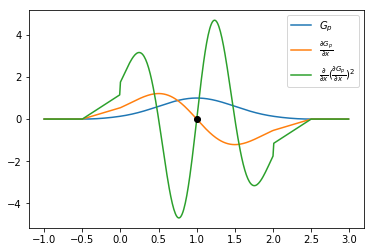As a reminder, what we are computing is how close to zero (squared value) the gradient with respect to the x-coordinate is as we move the atom along the x-axis at a specific grid point $G_p$ at $(1,0,0)$.

You can see that the second derivative is discontinuous when it switches to the quadratic form. I suspect we should probably switch to truncated gradients at $2r$ if we are optimizing for gradient values.

Note what we are plotting is the gradient with respect to the square of the gradients.

In :
plt.plot(xvals,y2vals,label=r'$\frac{\partial^2 G_p}{\partial x^2}$')
plt.plot(x+1,-128*x**3*np.exp(-4*x**2) + 32*x*np.exp(-4*x**2))

#plt.legend(loc='best');

Out:
[<matplotlib.lines.Line2D at 0x7f6ecbf0eb00>]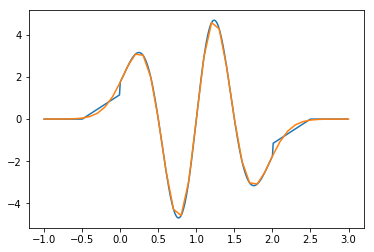At least in one dimension, looks pretty good.

I implemented truncated gradients - set the gaussian multiplier to negative and it won't have a quadratic part after the gaussian part.

In :
gmaker = molgrid.GridMaker(resolution=0.5,dimension=6.0,gaussian_radius_multiple=-2.0)

xvals = np.arange(-1.0,3,.01)
y2vals = []
y1vals = []
y0vals = []
for x in xvals:
coords = x  #evaluate y direction
outgrid = molgrid.Coords2GridFunction.apply(gmaker, (0,0,0), coords, types, radii)
gp = outgrid
y0vals.append(float(gp))
y1vals.append(float(Lg))
fancyL = torch.sum(Lg**2)
y2vals.append(val)

In :
plt.plot(xvals,y0vals,label='$G_p$')
plt.plot(xvals,y1vals,label=r'$\frac{\partial G_p}{\partial x}$')
plt.plot(xvals,y2vals,label=r'$\frac{\partial}{\partial x}(\frac{\partial G_p}{\partial x})^2$')

plt.plot(,,'ok')
plt.legend(loc='best');